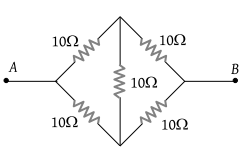# NEET Questions Solved

PMT - 2000

The effective resistance between points A and B is(1) 10 Ω

(2) 20 Ω

(3) 40 Ω

(4) None of the above three values

(1) This is a balanced Wheatstone bridge. Therefore no current will flow from the diagonal resistance 10 Ω

∴ Equivalent resistance $=\frac{\left(10+10\right)×\left(10+10\right)}{\left(10+10\right)+\left(10+10\right)}$ = 10 Ω

Difficulty Level:

• 82%
• 7%
• 4%
• 9%